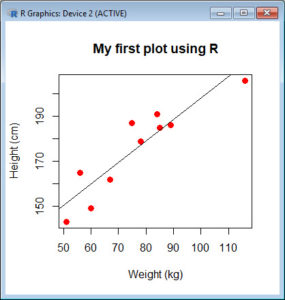In Part 1 we installed R and used it to create a variable and summarise it using a few simple commands. Today let’s re-create that variable and also create a second variable, and see what we can do with them.
As before, we take `height` to be a variable that describes the heights (in cm) of ten people. Copy and paste the following code to the R command line to create this variable.
`height = c(186, 165, 149, 206, 143, 187, 191, 179, 162, 185)`
Now let’s take `weight` to be a variable that describes the weights (in kg) of the same ten people. Copy and paste the following code to the R command line to create the `weight` variable.
`weight = c(89, 56, 60, 116, 51, 75, 84, 78, 67, 85)`
Both variables are now stored in the R workspace. To view them, enter:
```height weight```
We can now create a simple plot of the two variables as follows:
`plot(weight, height)`
However, this is a rather simple plot and we can embellish it a little. Copy and paste the following code into the R workspace:
`plot(weight, height, pch = 16, cex = 1.3, col = "red", main = "My first plot using R", xlab = "Weight (kg)", ylab = "Height (cm)")`
In the above code, the syntax `pch = 16` creates solid dots, while `cex = 1.3` creates dots that are 1.3 times bigger than the default (where `cex = 1`). More about these commands later.
Now let’s perform a linear regression on the two variables by adding the following text at the command line:
`lm(height ~ weight)`
We see that the intercept is 102.7071 and the slope is 0.9539.
Finally, we can add a best fit line to our plot by adding the following text at the command line:
`abline(102.7071, 0.9539)`
None of this was so difficult! 🙂
In Part 3 we will look again at regression and create more sophisticated plots.
David

#### Annex: R codes used

```# Creating the height variable
height = c(186, 165, 149, 206, 143, 187, 191, 179, 162, 185)

# Creating the weight variable
weight = c(89, 56, 60, 116, 51, 75, 84, 78, 67, 85)

# Show content of both variables
height
weight

# Create a graph (scatterplot) for two variables
plot(weight, height)

# Improved scatterplot for two variables
plot(weight, height, pch = 16, cex = 1.3, col = "red", main = "My first plot using R", xlab = "Weight (kg)", ylab = "Height (cm)")

# Estimating the simple linear regression
lm(height ~ weight)

# Adding regression line on the existing graph
abline(102.7071, 0.9539)
```

Screenshots of the R console with all results: